C++ Programs to Check Palindrome String

In this tutorial you will learn about the C++ Programs to Check Palindrome String and its application with practical example.

In this tutorial, we will learn to create a c++ program that  checks Palindrome String  using c++ programming.

Prerequisites

Before starting with this tutorial we assume that you are best aware of the following C ++ programming topics:

• Operators.
• Basic input/output.
• String
• mathematical functions.
• in-built functions.

What Is Palindrome of String?

A palindrome of a String that reads the same backward and forward.

Example : dad , nayan , pop, abcba and  etc…

Output

Palindrome string.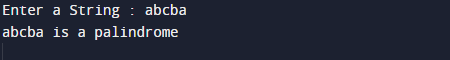Not a Palindrome string.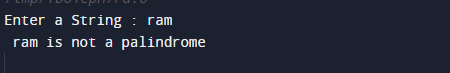In the above program, we have first declared and initialized a set variables required in the program.

• strng =it will hold given string.
• i= for iteration.
• cmp= for holding true false value.
• len = it will hold length of string.

In the next statement user will be prompted to enter a string which will be assigned to variable ‘strng’.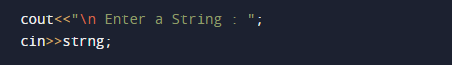after that with the help of strlen() function we find the length of string.

Now for loop iterator upto ‘len’ number of terms. Inside the loop we compare string.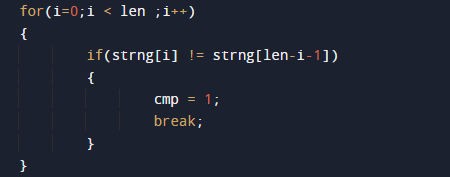First character in the strng is the same as last character in the same string.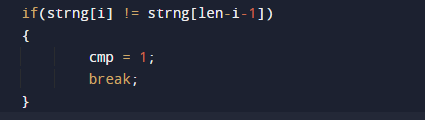is the first character is the same as ‘len-i-1’th character and  so on .This process continues till length of a string .If any above condition fails, the cmp varibale is set to  cmp=1 which is  true(1), which implies that the string is not a palindrome.If above condition satisfied, the cmp variable is all ready  set to  cmp=0 which is  false(0), which implies that the string is a palindrome.In this tutorial we have learn about the C++ Programs to Check Palindrome String and its application with practical example. I hope you will like this tutorial.